# DAV Class 3 Maths Chapter 4 Worksheet 8 Solutions

The DAV Class 3 Maths Book Solutions and DAV Class 3 Maths Chapter 4 Worksheet 8 Solutions of Multiplication offer comprehensive answers to textbook questions.

## DAV Class 3 Maths Ch 4 WS 8 Solutions

Question 1.
In a book, there are 117 pages. How many pages are there in 21 such books?
Solution:
Pages in a book = 117
No. of books = 21
No. of pages = 117 × 2121 books have 2457 pages.

Question 2.
Find the cost of 19 chairs, if each chair costs 290.
Solution:
Cost of one chair = 290
Cost of 19 chairs = 290 × 19
= ₹ 5510cost of 19 chairs is ₹ 5510.Question 3.
A necklace contains 108 beads. How many beads will 32 such necklaces contain?
Solution:
No. of beads in 1 necklace = 108
No. of beads in 32 necklaces = 108 × 32 = 3456Question 4.
There are 32 balloons in one packet. How many balloons 24 such packets?
Solution:
1 packet contains = 32 balloons
24 packets contains = 32 × 24 = 768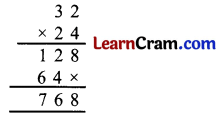768 balloons.

Question 5.
In a hail, there are 35 rows of chairs. Each row has 15 chairs. How many chairs are there in the hail?
Solution:
No. of chairs in 1 row = 15
No. of chairs in 35 rows = 15 × 35 = 525525 chairs are there in the hail.### DAV Class 3 Maths Chapter 4 Value Based Questions

Question 1.
Radha and her family birthday happily.

Solution:
So she spend = 36 × 65
= ₹ 2340

2. Suggest any two ways in which you can help the children of an orphanage.
Solution:
(i) By giving books, clothes to them.
(ii) By making them a part in our festivals like birthday, independenceday and diwali etc.

3. Suggest some items which can be given as return gifts without purchasing them.
Solution:
Hand-made cards, pencil stand, flowers etc.Question 1.
Multiply:

(i) 324 × 2
Solution:
324 × 2(ii) 703 × 2
Solution:
703 × 2(iii) 506 × 7
Solution:
506 × 7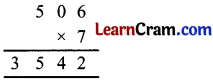(iv) 806 × 22
Solution:
806 × 22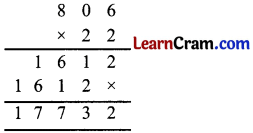(v) 8012 × 0
Solution:
8012 × 0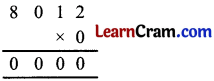(vi) 526 × 24
Solution:
526 × 24(vii) 726 × 48
Solution:
726 × 48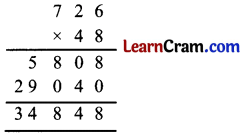(viii) 103 × 28
Solution:
103 × 28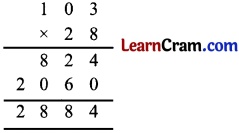Question 2.
Solve the puzzles.

(i)Solution: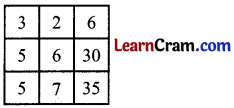(ii)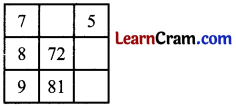Solution:Question 3.
Fill in the blanks.

(i) 12 × 2 = ____
Solution:
12 × 2 = 24

(ii) 15 × 5 = ____
Solution:
15 × 5 = 75

(iii) 12 × ____ = 60
Solution:
12 × 5 = 60

(iv) 11 × ____ = 55
Solution:
11 × 5 = 55

(v) 15 × ____ = 75
Solution:
15 × 5 = 75

(vi) 10 × 10 = ____
Solution:
10 × 10 = 100

(vii) 9 × ____ = 72
Solution:
9 × 8 = 72

(viii) 5 × ____ = 50
Solution:
5 × 10 = 50Question 4.
Using properties of multiplication solve the following:

(i) 29 × 0 = ____
Solution:
29 × 0 = 0

(ii) 76 × 1 = ____ × 76
Solution:
76 × 1 = 1 × 76

(iii) 58 × 70 = 58 × ____ × 10
Solution:
58 × 70 = 58 × 7 × 10

(iv) 39 × 1 = 1 × ____
Solution:
39 × 1 = 1 × 39

(v) 1050 × 0 = ____
Solution:
1050 × 0 = 0Question 5.
47 Children sent 20 New Year cards each. How many cards did they send in all?
Solution:
1 children sent cards = 20
47 children sent cards = 20 × 47
= 940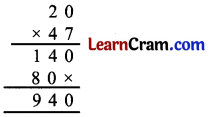940 cards.

Question 6.
A man buys 36 bananas for his monkeys every day. How many bananas does he buy in 2 months?
Solution:
No. of bananas in 1 day = 36
No. of bananas in 2 × 30 days
= 36 × 60
= 2160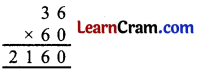2160 bananas.### DAV Class 3 Maths Chapter 4 Worksheet 8 Notes

Question 1.
A box has a dozen eggs. How many eggs do 35 such boxes have? 3 5
Solution:
No. of eggs in 1 box = 12
Number of eggs in 35 boxes = 12 × 35 = 42035 boxes have 420 eggs.